Function Repository Resource:

# EdgeChromaticNumber

Compute the smallest number of colors needed in a proper edge coloring of a graph

Contributed by: Wolfram Staff (original content by Sriram V. Pemmaraju and Steven S. Skiena)
 ResourceFunction["EdgeChromaticNumber"][g] gives the minimum number of colors necessary for proper edge coloring of the graph g.

## Details and Options

A proper edge coloring is an assignment of colors to edges of the graph such that no two adjacent edges share the same color.
The edge chromatic number is also called the chromatic index.
ResourceFunction["EdgeChromaticNumber"] works with undirected graphs, directed graphs, multigraphs and mixed graphs, treating directed edges as undirected ones.

## Examples

### Basic Examples (3)

Get a sample graph:

 In:=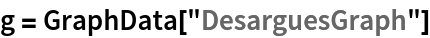Out=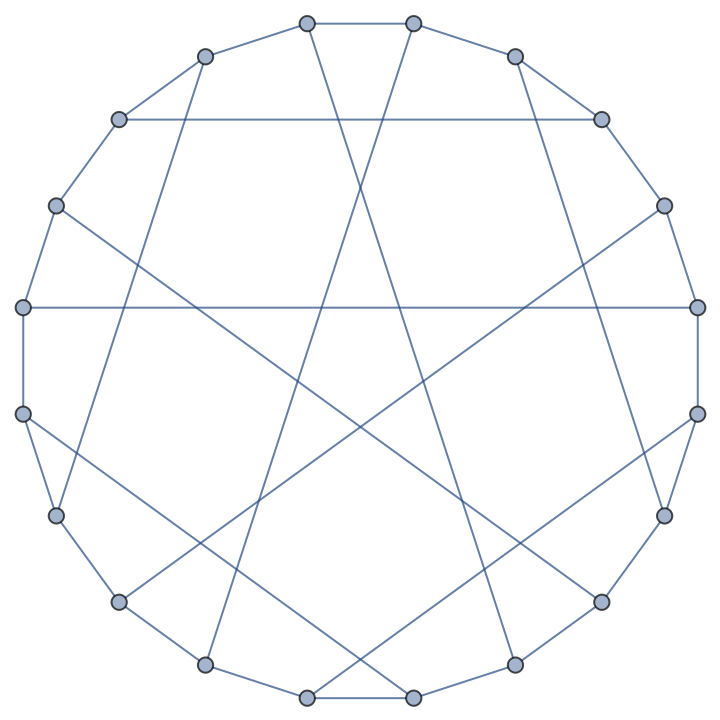Compute the minimum number of colors needed to color the edges of the graph so that no two adjacent edges have the same color:

 In:=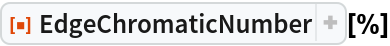Out=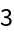Show a proper edge coloring of the graph:

 In:=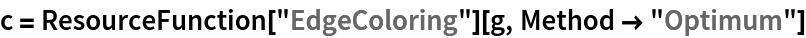Out=In:=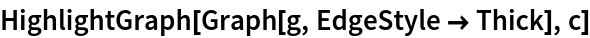Out=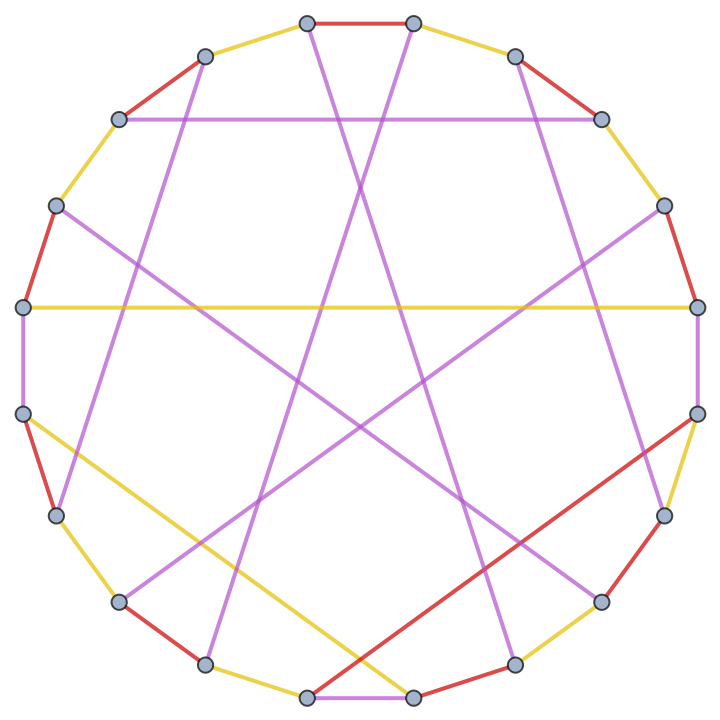In:=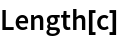Out=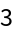### Scope (4)

EdgeChromaticNumber works with undirected graphs:

 In:=Out=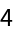Directed graphs:

 In:=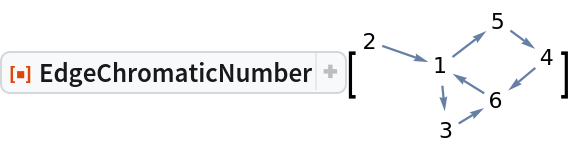Out=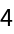Multigraphs:

 In:=Out=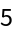Mixed graphs:

 In:=Out=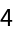### Properties and Relations (6)

For named graphs, the edge chromatic numbers can be obtained using GraphData:

 In:=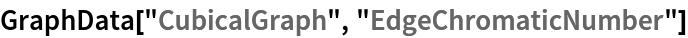Out=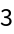Compute directly:

 In:=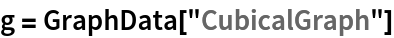Out=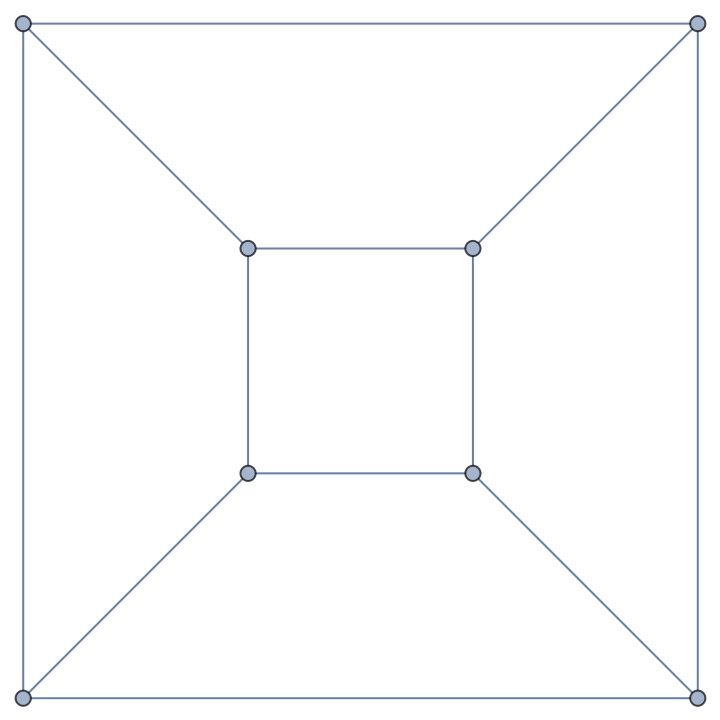In:=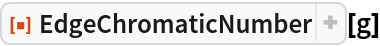Out=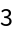The edge chromatic number of a simple graph is at least the maximum vertex degree of the graph and no more than the maximum degree plus one (Vizing's theorem):

 In:=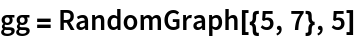Out=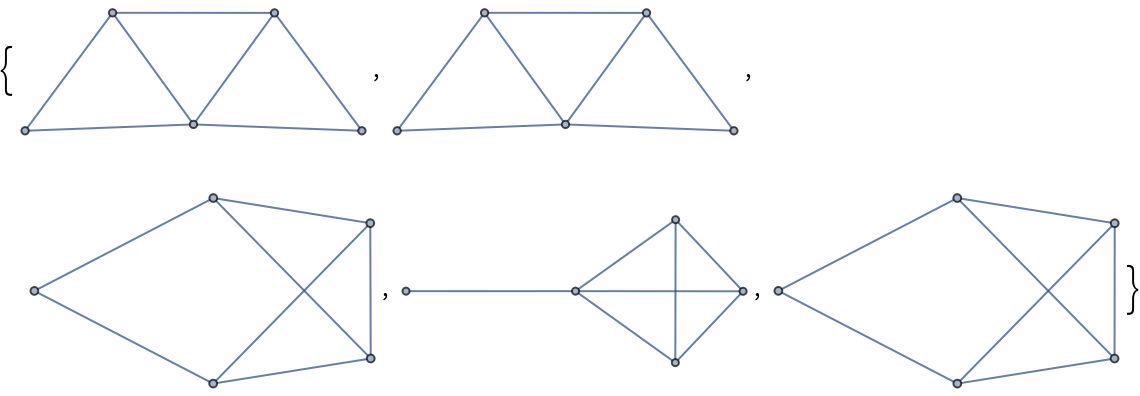In:=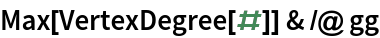Out=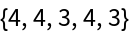In:=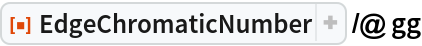Out=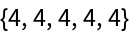The edge chromatic number is exactly the maximum vertex degree of the graph for class-1 graphs, including king graphs:

 In:=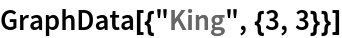Out=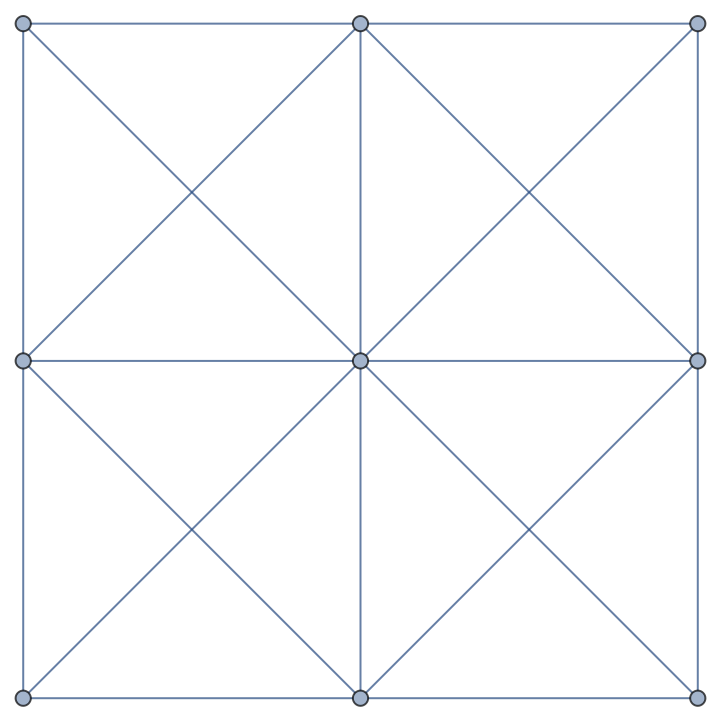In:=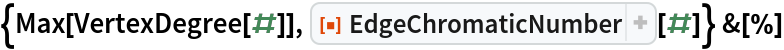Out=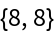Bipartite graphs are class-1 graphs:

 In:=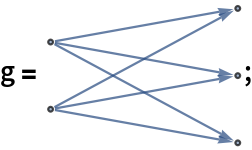In:=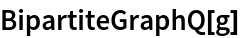Out=In:=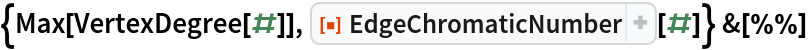Out=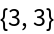The edge chromatic number is 1 plus the maximum vertex degree of the graph for class-2 graphs, including Petersen graphs:

 In:=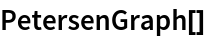Out=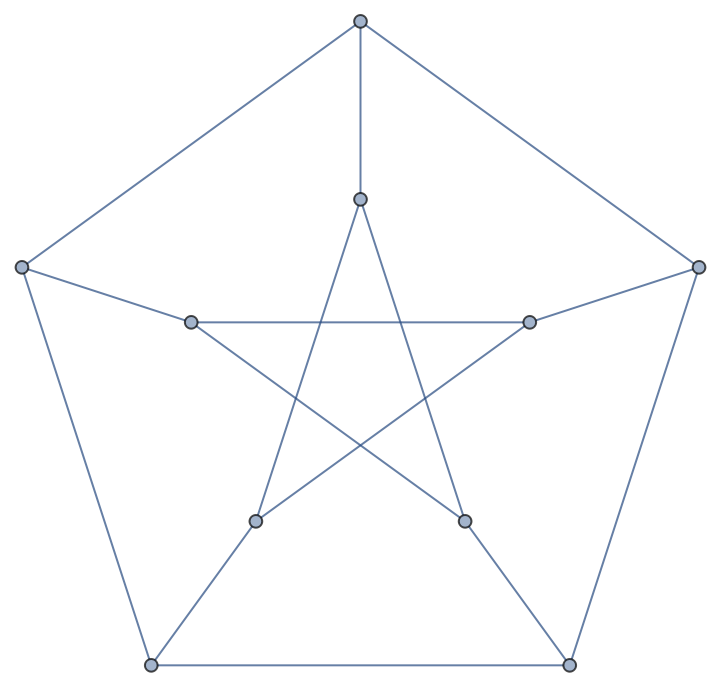In:=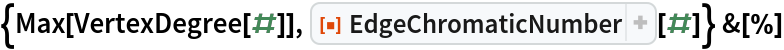Out=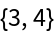Vizing's theorem does not hold for multigraphs:

 In:=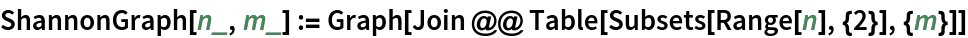In:=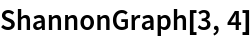Out=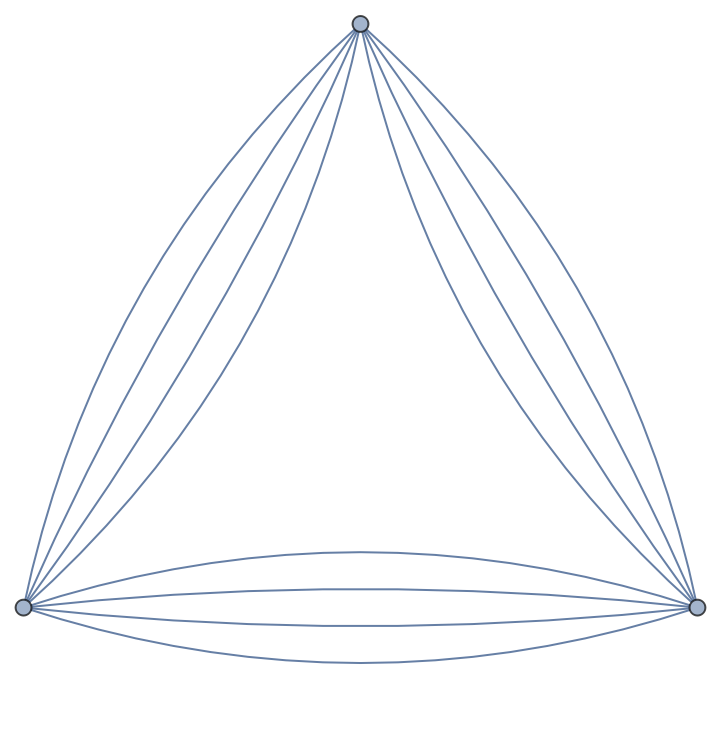In:=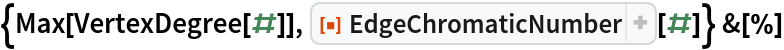Out=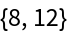## Version History

• 1.0.0 – 22 October 2020